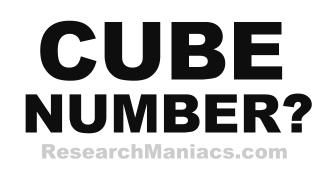Is 30 a cube number?

Cube Question: Is 30 a cube number?

For 30 to be a cube number the following equation must be true:

n3 = 30 where n is a whole number.

In other words, if n*n*n = 30, then 30 is a cube number.

There are no whole numbers you can set to n to make the equation above 30. Therefore, we can conclude that 30 is NOT a cube number.

Answer: Is 30 a cube number? = NO

 Cube Number? Check other numbers to see if they are cube. List of Cube Numbers Here is a list of cube numbers.